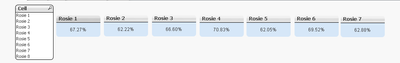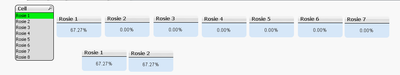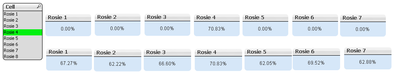# QlikView App Dev

Discussion Board for collaboration related to QlikView App Development.

Announcements
Our next Qlik Insider session will cover new key capabilities. Join us August 11th REGISTER TODAY
cancel
Showing results for
Did you mean:Creator II

## Set Analysis With Variable

Hi All,

I have created some text boxes based on the Cell Field Values. Below is the expression.

=Num(Sum(Aggr(If(Cell = 'Rosie 1', \$(vOEE)),Cell)),'#,##0.00%')

Without Selection Cell Field Selection values are showing in all text boxes.if i select one value from Cell field, selected value is showing in text boxes where as remaining its showing as 0.My requirement is whatever value selected from Cell , all text boxes should be showing their corresponding values.

Labels (1)
• ### Application Development

1 Solution

Accepted SolutionsMVP

Hi Dinesh,

Try like belowNum(\$(vAvailability(\$1)) * \$(vPerformance(\$1)) * \$(vQuality(\$1)),'#,##0.00%')

Thanks & Regards,
Mayil Vahanan R
8 RepliesMVP

maybe something like

``Num(Sum({\$<Cell={'Rosie 1'}>} \$(vOEE)),'#,##0.00%')``

what is the content of variable vOEE?

hope this helps

regards

MarcoCreator II
Author

Hi Macro,

vOEE is

Num(If(Sum([Net Available]) <> 0, Sum(Running)/Sum([Net Available]), 0),'#,##0.00%') *

Num(If(Sum([Pieces per Minute]) * Sum(Running) <> 0, Sum([Actual Production (Pieces)])/(Sum([Pieces per Minute]*Running)),0),'#,##0.00%') *

Num(If(Sum([Actual Production (Pieces)] ) <> 0, (Sum([Actual Production (Pieces)]) - Sum(Reject))/Sum([Actual Production (Pieces)]),0),'#,##0.00%')

Note: I want to extract the particular value from the variable. But we can use set expression in the above formula.

Regards,

DineshMVP

HI Dinesh,

Change the vOEE  like below

Num(If(Sum({<\$1>}[Net Available]) <> 0, Sum({<\$1>}Running)/Sum({<\$1>}[Net Available]), 0),'#,##0.00%') *

Num(If(Sum({<\$1>}[Pieces per Minute]) * Sum({<\$1>}Running) <> 0, Sum({<\$1>}[Actual Production (Pieces)])/(Sum({<\$1>}[Pieces per Minute]*Running)),0),'#,##0.00%') *

Num(If(Sum({<\$1>}[Actual Production (Pieces)] ) <> 0, (Sum({<\$1>}[Actual Production (Pieces)]) - Sum({<\$1>}Reject))/Sum({<\$1>}[Actual Production (Pieces)]),0),'#,##0.00%')

Try like below

=\$(vOEE(Cell={'Rosie 1'}))

Thanks & Regards,
Mayil Vahanan RCreator II
Author

Hi MayilVahanan,

I have tried your logic but its showing without any selection overall OEE.If i Select the value from Cell as Rosie 1 both values are same it means for Rosie 2 showing same value.

Rosie 1 Text Box: =\$(vOEE(Cell = {'Rosie 1'}))

Rosie 2 Text Box: =\$(vOEE(Cell = {'Rosie 2'})) but the real value is 62.22% as given the above image.

Thanks & Regard,

Dinesh.MVP

Hi Dinesh

Can you send  the sample file..

Thanks & Regards,
Mayil Vahanan RCreator II
Author

Hi,

PFA...MVP

Hi Dinesh,

Try like belowNum(\$(vAvailability(\$1)) * \$(vPerformance(\$1)) * \$(vQuality(\$1)),'#,##0.00%')

Thanks & Regards,
Mayil Vahanan RCreator II
Author

Hi MayilVahanan,

Its working fine.Thanks & Regards,

Dinesh Kumar.Community Browser